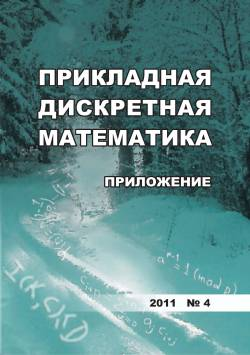# Дискретный логарифм для нильпотентных групп и криптанализ полилинейной криптографической системы

We present an efficient algorithm to compute a discrete logarithm in a finite nilpotent group, or more generally, in a finitely generated nilpotent group. Special cases of a finite p-group (p is a prime) and a finitely generated torsion free nilpotent group are considered. Then we show how the derived algorithm can be generalized to an arbitrary finite or finitely generated nilpotent group respectively. We suppose that group is presented by generating elements and defining relators or as a subgroup of a triangular matrix group over a prime finite field (in finite case) or over the ring of integers (in torsion-free case). On the base of the derived algorithm we give a cryptanalysis of some schemes of polylinear cryptography known in the literature.

## Ключевые слова

discrete logarithm, nilpotent group, polylinear system, cryptanalysis

## Авторы

 ФИО Организация Дополнительно E-mail Романьков Виталий Анатольевич Омский государственный университет им. Ф.М. Достоевского доктор физико-математических наук, профессор, заведующий кафедрой romankov48@mail.ru
Всего: 1

## Ссылки

Menezes A. J., van Oorchot P. C., and Vanstone S. A. Handbook of Applied Cryptography. N.Y., CRC Press, 1997.
Koblitz N. A Course in Number Theory and Cryptography. N.Y., Springer, 1987.
Roman'kov V. A. Vvedenie v kriptografiyu [Introduction to Cryptography]. Moscow, Forum Publ., 2012 (in Russian).
Shor P. Polynomial-time algorithm for prime factorization and discrete logarithms on a quantum computer. SIAM J. Comput., 1997, no. 5, pp. 1484-1509.
Grover L. K. A fast quantum mechanical algorithm for database search. Proc. 28th Ann. ACM Symp. on Theory of Comput., 1997, no. 5, pp. 212-219.
Myasnikov A., Shpilrain V., and Ushakov A. Group-Based Cryptography. Barselona-Basel, CRM, 2008 (Advances Courses in Math.).
Myasnikov A., Shpilrain V., and Ushakov A. Non-commutative Cryptography and Complexity of Group-Theoretic Problems. With Appendix by Natalia Mosina. Math. Surveys and Monographs, 2011, vol. 177, Providence RI, AMS.
Roman'kov V. A. Algebraicheskaya kriptografiya [Algebraic cryptography]. Omsk, OmSU Publ., 2013. (in Russian)
Myasnikov A. and Roman'kov V. A linear decomposition attack. Groups, Complexity, Cryptology, 2015, vol. 7, pp. 81-94.
Roman'kov V. A non-linear decomposition attack. Groups, Complexity, Cryptology, 2015, vol. 8, pp. 197-207.
Roman'kov V. A. Essays in Algebra and Cryptology. Algebraic Cryptanalysis. Omsk, OmSU Publ., 2018.
Tsaban B. Polynomial-time solutions of computational problems in noncommutative-algebraic cryptography. J. Cryptology, 2015, vol.28, pp. 601-622.
Ben-Zvi A., Kalka A., and Tsaban B. Cryptanalysis via algebraic spans. LNCS, 2018, vol.109991, pp. 1-20.
Kargapolov M. I. and Merzlyakov Y. I. Fundamentals of the Theory of Groups. N.Y., Springer Verlag, 1979.
Hall P. Nilpotent Groups. Edmonton Notes on Nilpotent Groups. Queen Mary College Math. Notes Math. Dept., London, Queen Mary College, 1969.
Lennox J. C. and Robinson D. J. S. The Theory of Infinite Soluble Groups. Oxford, Clarendon Press, 2004 (Oxford Math. Monographs).
Roman'kov V. A. and Khisamiev N. G. Nil'potentnye grruppy [Nilpotent Groups]. Ust-Kamenogorsk, EKSTU Publ., 2013. (in Russian)
Menezes A. J. and Vanstone S. A. A note on cyclic groups, finite fields and discrete logarithm problem. AAECC, 1992, vol. 3, pp. 67-74.
Boneh D. and Silverberg A. Applications of multilinear forms in cryptography. Contemporary Math., 2003, vol.324, pp. 71-90.
Lin H. and Tessaro S. Indistinguishability Obfuscation from Trilinear Maps and Block-Wise Local PRGs. Cryptology ePrint Archive, Report 2017/250, 2017. https://eprint.iacr.org/ 2017/250
Huang M. A. Trilinear Maps for Cryptography. arXiv: 1803.10325, 2018.
Mahalanobis A. The Diffie - Hellman key exchange protocol and non-abelian nilpotent groups. Israel J. Math., 2008, vol.165, pp. 161-187.
Kahrobaei D., Tortora A., and Tota M. Multilinear Cryptography Using Nilpotent Groups. arXiv: 1902.08777v1 [cs. CR] 23 Feb 2019. 8 p.